## Example Questions

← Previous 1

### Example Question #8 : Integers

Which of the following is a prime number?

15

71

9

6

51

71

Explanation:

a prime number is divisible by itself and 1 only

list the factors of each number:

6: 1,2,3,6

9: 1,3,9

71: 1,71

51: 1, 3,17,51

15: 1,3,5,15

### Example Question #9 : Integers

If x is a prime number, then 3x is

cannot be determined

odd

even

a prime number

divisible by 4

cannot be determined

Explanation:

Pick a prime number to see that 3x is not always even, for example 3 * 3 = 9.

But 2 is a prime number as well, so 3 * 2 = 6 which is even, so we can't say that 3x is either even or odd.

Neither 9 nor 6 in our above example is prime, so 3x is not a prime number.

Lastly, 9 is not divisible by 4, so 3x is not always divisible by 4.

Therefore the answer is "Cannot be determined".

### Example Question #1 : Factors / Multiples

Which of the following pairs of numbers are twin primes?

1, 3

3, 5

1, 2

13, 19

2, 3

3, 5

Explanation:

For starters, 1 is not a prime number, so eliminate the answer choices with 1 in them. Even if you have no idea what twin primes are, at least you've narrowed down the possibilities.

Twin primes are consecutive prime numbers with one even number in between them. 3 and 5 is the only set of twin primes listed. 2 and 3 are not separated by any numbers, and 13 and 19 are not consecutive primes, nor are they separated by one even number only. You should do your best to remember definitions and formulas such as this one, because these questions are considered "free" points on the test. There is no real math involved, just something to remember! Being able to answer a question like this quickly will give you more time for the computationally advanced problems.

### Example Question #1 : How To Find Out If A Number Is Prime

What must be true of all prime numbers?

Every positive prime has a corresponding negative prime.

The sum of two primes is always even.

Multiplying two primes will always produce an odd number.

The distribution of primes is random.

There are only two primes that are consecutive positive integers on the number line.

There are only two primes that are consecutive positive integers on the number line.

Explanation:

Let's go through the five statements.

The sum of two primes is always even: This is only true of the odd primes. 2 is also a prime number, however, and 2 plus an odd number is odd.

Every positive prime has a corresponding negative prime: This is also false. There are no negative primes. A prime number is defined as a number greater than 1 that is divisible by only 1 and itself.

There are only two primes that are consecutive positive integers on the number line: This is true and therefore the correct answer. 2 and 3 are the only primes that are consecutive. Because 2 is the only even prime, all other primes must have at least one number in between them (since every two odd numbers are separated by an even).

Multiplying two primes will always produce an odd number: This is also only true of odd primes. 2 * odd prime = even.

The distribution of primes is random: False. The primes are logarithmically distributed.

### Example Question #1 : Prime Numbers

Quantitative Comparison

Quantity A: The smallest prime number multiplied by 3 and divided by the least common multiple of 5 and 10

Quantity B: The smallest odd prime number multiplied by 2 and divided by the 2nd smallest odd prime

Quantity A is greater.

The two quantities are equal.

The relationship cannot be determined from the information given.

Quantity B is greater.

Quantity B is greater.

Explanation:

Quantity A: The smallest prime number is 2. We also need the least common multiple of 5 and 10, which is 10.

So Quantity A = 2 * 3 / 10 = 3/5

Quantity B: The smallest odd prime is 3. The second smallest odd prime is 5.

So Quantity B = 3 * 2 / 5 = 6/5

Quantity B is greater.

### Example Question #3 : How To Find Out If A Number Is Prime

Quantitative Comparison

Quantity A: The number of prime numbers between 0 and 100, inclusive.

Quantity B: The number of prime numbers between 101 and 200, inclusive.

The two quantities are equal.

Quantity B is greater.

Quantity A is greater.

The relationship cannot be determined from the information given.

Quantity A is greater.

Explanation:

As we go up on the number line, the number of primes decreases almost exponentially. Therefore there are far more prime numbers between 0 and 100 than there are between 101 and 200. This is a general number theory point that is important to know, but trying to come up with some primes in these two groups will also quickly demonstrate this principle.

### Example Question #4 : How To Find Out If A Number Is Prime

Which number is greater than the sum of all the prime factors of 330?Explanation:

The prime factorization of 330 is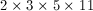.

The sum of the prime factors is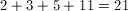.

23 is the only answer choice greater than 21.

### Example Question #1 : How To Find Out If A Number Is Prime

A prime number is divisible by:

ItselfIt depends on the prime number.

Explanation:

The definition of a prime number is a number that is divisible by only one and itself. A prime number can't be divided by zero, because numbers divided by zero are undefined. The smallest prime number is 2, which is also the only even prime.

### Example Question #6 : How To Find Out If A Number Is Prime

What is half of the third smallest prime number multiplied by the smallest two digit prime number?

This number does not exist.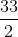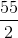Explanation:

The third smallest prime number is 5. (Don't forget that 2 is a prime number, but 1 is not!)

The smallest two digit prime number is 11.

Now we can evaluate the entire expression: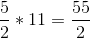### Example Question #7 : How To Find Out If A Number Is Prime

a, b and c are integers, and a and b are not equivalent.

If ax + bx = c, where c is a prime integer, and a and b are positive integers which of the following is a possible value of x?

1

4

2

3

1

Explanation:

This question tests basic number properties. Prime numbers are numbers which are divisible only by one and themselves. Answer options '2' and '4' are automatically out, because they will always produce even products with a and b, and the sum of two even products is always even. Since no even number greater than 2 is prime, 2 and 4 cannot be answer options. 3 is tempting, until you remember that the sum of any two multiples of 3 is itself divisible by 3, thereby negating any possible answer for c except 3, which is impossible. There are, however, several possible combinations that work with x = 1. For instance, a = 8 and b = 9 means that 8(1) + 9(1) = 17, which is prime. You only need to find one example to demonstrate that an option works. This eliminates the "None of the other answers" option as well.

← Previous 1

Tired of practice problems?

Try live online GRE prep today.